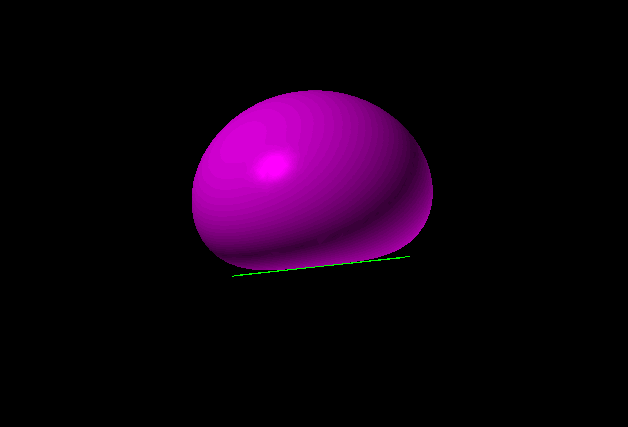### Diffuse or Lambertian Reflection

When a photon hits a rough surface, or a gas molecule hits just about any surface, it rebounds in a direction not much related to its incoming direction. We talk here about the case where photons or molecules reflect in a statistically independent direction. When the new direction is statistically independent of the old, the new direction has a very specific distribution. This is called Lambertian reflection and applies to ray tracing with diffuse surfaces, and many MonteCarlo physics calculations.

We present an easy calculation to efficiently choose such a direction for the rebounding photon or molecule.

### First some Physics

I will baldly assert for now that if the direction of the rebounding photon is statistically independent of its incoming direction then Lambert’s cosine law must apply to preserve the second law of thermodynamics. The argument posits a region bounded by the surface where radiation is at equilibrium. The photon flux thru any small planar loop is proportional to the loop’s area, and is independent of the loop’s location and orientation, even if the loop is very near the surface. A particular photon does not know if the system is at equilibrium and so bounces with the same statistics all the time.

### Monte Carlo Calculation

Suppose that we have a pipe connecting two low pressure chambers and we want to know how fast the pipe will equalize the pressures. The pressure is so low that molecules don’t run into each other, but only the walls of the chambers and the sides of the pipe. The pipe is one inch in diameter and two inches long. Some of the particles that enter the pipe will bounce off the side of the pipe so as to leave the same end of the pipe that it entered. Others will make their way thru. What is the ratio?

In ray tracing one might do a similar calculation with photons. If several rays per pixel are used then the surface noise may be reduced. This requires similar calculations.

A common problem is to choose new directions for a rebounding photon or molecule. The only input to the problem is the orientation of the surface and a source of random numbers.

### What is the distribution?

The set of possible directions forms a hemisphere of directions. We must select a vector for the new velocity. It turns out that the magnitude of the vector is irrelevant in both kinds of problem. Lambert claimed that the probability of the direction laying in some element of solid angle was proportional to the size of the element (naturally) but also the cosine of the angle between the direction and the normal to the surface. This makes it so that the brightness of the surface viewed head on, and from an oblique angle, are the same. (The second law demands this!) The naïve way to calculate this involves computing a cosine about twice per selected vector. Here is a faster way.

There is a 3D shape that I will call the squat sphere, SS, for its shape.It is the rotation about the z-axis of a curve in the x-z plane whose formula is (x2 + z2)2 = z. All z values are positive. It is a near sphere which goes thru the origin but is flat there. The three dimensional formula is (x2+y2+z2)2 = z.

The box [−.7, .7] × [−.7, .7] × [0, 1] contains SS with little room to spare. A randomly selected point from the box is chosen but then rejected unless it falls also in the SS, i.e. unless (x2+y2+z2)2 < z. This process selects random points uniformly throughout the interior of SS. The distribution of directions of these points from the origin is just the distribution we need assuming that the particle is rebounding from the x-y plane. No cosines or square roots need be computed. The SS occupies about 1/2 the volume of the box. About 6 random numbers are thus required on the average.

You might be convinced by reading and running this C program, which generates such samples and forms a histogram of the cosines of the resulting angles.